# Markov property

(diff) ← Older revision | Latest revision (diff) | Newer revision → (diff)

for a real-valued stochastic process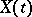,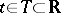The property that for any set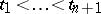of times fromand any Borel set,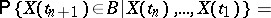(*)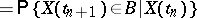with probability 1, that is, the conditional probability distribution of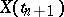given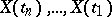coincides (almost certainly) with the conditional distribution ofgiven. This can be interpreted as independence of the "future"and the "past"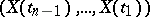given the fixed "present"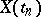. Stochastic processes satisfying the property (*) are called Markov processes (cf. Markov process). The Markov property has (under certain additional assumptions) a stronger version, known as the "strong Markov property" . In discrete timethe strong Markov property, which is always true for (Markov) sequences satisfying (*), means that for each stopping time(relative to the family of-algebras,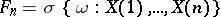), with probability one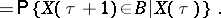How to Cite This Entry:
Markov property. Encyclopedia of Mathematics. URL: http://encyclopediaofmath.org/index.php?title=Markov_property&oldid=11571
This article was adapted from an original article by A.N. Shiryaev (originator), which appeared in Encyclopedia of Mathematics - ISBN 1402006098. See original article